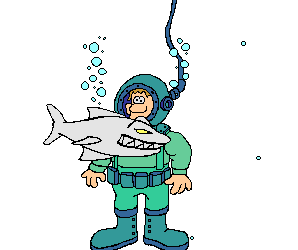Prealgebra Proportion Word Problems 1. If 6 pounds of apples cost \$9, then how much would 21 pounds of apples cost? 2. If \$24 worth of fertilizer covers 5,000 square feet, then how much would it cost to cover 30,000 square feet? 3. If a chain link fence costs \$180 for 20 feet installed, how much would it cost to install 300 feet? 4. The scale on a map is 1 inch equals 5 feet. What is the distance between two points on the map that are 8 1/2 inches apart on the map? 5. A \$4 sales tax is charged for a \$50 purchase. At this rate, what is the sales tax for a \$1200 purchase? 6. A store makes a profit of \$15,000 for every 300 coats that they sell. If they make a profit of \$25,000, how many coats did they sell?